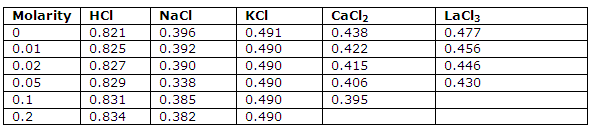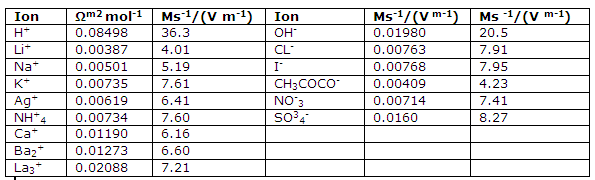How to calculate solutions ionic mobilities?

Transference numbers and molar conductors can be used to calculate ionic mobilities.

This tables under is giving the transference numbers for positive ions at 25 degree C and the values obtained by extrapolation to infinite dilution:Molar ionic conductances and ionic mobilities at infinite dilution and 25 degree C.Values can now be obtained for the contributions the individual ions of an electrolyte make to the molar conductance. The empirical law of Kohlrausch implies that a infinite dilution the molar conductance can be interpreted in terms of such ionic contributions and that the contributions of an ion are independent of the other ion of the electrolyte. At infinite dilution, therefore, we wrote:

?° = v + λ°+ v- λ°- where

λ°+ and λ°- are the molar ionic conductors at infinite dilution. Since the transference numbers give the fraction of the total current by each ion, i.e., the fraction of the total conductance that each contributes, we can write;

v+ λ°+ = t°+ ?°  and v- λ°- = t°- ?°

where t°+ and t°- are the transference numbers extrapolated to infinite dilution.

Ionic mobilities: consider a cell of the type used to introduce the concept of molar conductance. Such a cell consists of two electrodes 1m apart and of cross-section area A such that an amount of solution that contains 1 mol of electrolyte is held between the electrodes. For an applied voltage , a current I will flow through the cell. These electrical quantities are related, since the conductance of such a cell is the molar conductance of the electrolyte, by:

I = ∫/R or I = ?∫

At infinite dilution the current can be attributed to the independent flow of positive and negative ions, and one can write:

I = ?°∫ = [v+ λ°+ + v- λ°- ] ∫ = v + λ°+ ∫ + v- λ°- ∫ = I+ + I-

#### Related Questions in Chemistry

• ##### Q :Unit of mole fraction Provide solution

Provide solution of this question. Unit of mole fraction is: (a) Moles/litre (b) Moles/litre2 (c) Moles-litre (d) Dimensionless

• ##### Q :Question based on relative lowering of

Give me answer of this question. When a non-volatile solute is dissolved in a solvent, the relative lowering of vapour pressure is equal to: (a) Mole fraction of solute (b) Mole fraction of solvent (c) Concentration of the solute in grams per litre (d) Concentratio

• ##### Q :Solubility are halides are halogens

are halides are halogens more soluble? why?

• ##### Q :Question 6 A student was analyzing an

A student was analyzing an unknown containing only Group IV cations. When the unknown was treated with 3M (NH4)2CO3 solution, a white precipitate formed. Because the acetic acid bottle was empty, the student used 6M HCl to dissolve the precipitate. Following the procedure of this experiment, the stu

• ##### Q :Vapour pressure over mercury Choose the

Choose the right answer from following. At 300 K, when a solute is added to a solvent its vapour pressure over the mercury reduces from 50 mm to 45 mm. The value of mole fraction of solute will be: (a)0.005 (b)0.010 (c)0.100 (d)0.900

• ##### Q :Statement of Henry law Determine the

Determine the correct regarding Henry’s law: (1) The gas is in contact with the liquid must behave as an ideal gas (2) There must not be any chemical interaction among the gas and liquid (3) The pressure applied must be high (4) All of these.

• ##### Q :Non-ideal Gases Fugacity The fugacity

The fugacity is a pressure like quantity that is used to treat the free energy of nonideal gases.

Now we begin the steps that allow us to relate free energy changes to the equilibrium constant of real, nonideal gases. The thermodynamic reaction

• ##### Q :Problem associated to vapour pressure

Provide solution of this question. 60 gm of Urea (Mol. wt 60) was dissolved in 9.9 moles, of water. If the vapour pressure of pure water is P0 , the vapour pressure of solution is:(a) 0.10P0 (b) 1.10P0 (c) 0.90P0 (d) 0.99P0

• ##### Q :Henry law question Answer the following

Answer the following qustion. The definition “The mass of a gas dissolved in a particular mass of a solvent at any temperature is proportional to the pressure of gas over the solvent” is:

(i) Dalton’s Law of Parti

• ##### Q :Equimolar solutions Select the right

Select the right answer of the question. Equimolar solutions in the same solvent have : (a)Same boiling point but different freezing point (b) Same freezing point but different boiling poin (c)Same boiling and same freezing points (d) Different boiling and differe